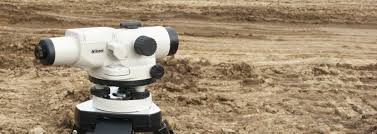Leveling may be the art of determining the relative heights or elevations of points are objects on the earth’s surface. It deals with measurements in a vertical plane.

Definitions of terms used in Leveling:

A level surface:

It is any surface parallel to the mean spheroidal surface of the earth e.g. surface of a still lake. Since the earth is a oblate spheroid, a level surface may be regarded as a curved surface, every point on which is equidistant from the center of the earth. It is normal to the plumb line at all points.

A level line:

It is line lying in a level surface.  It is therefore, normal to the plumb line at all points.

A horizontal plane:

It is a plane tangential to the level surface at that point. It is perpendicular to direction of gravity (plumb line).

A horizontal line:

It is any line lying in the horizontal plane. It is a straight line tangential to a level line.

A vertical line:

It is a line normal to the level surface through that point e. g. a plumb line.

A vertical plane:

It is a plane containing a vertical line.

A vertical angle:

Angle between two intersecting lines in a vertical plane, one of the two lines is commonly taken as horizontal in surveying.

A datum surface or line:

It is any arbitrarily assumed level surface or line from which vertical distances are measured in India the datum adopted for G.T.S. bench marks is the mean sea level at Karachi now in Pakistan.

The elevation:

It is vertical distance of a point above or below the datum. It is also known as the reduced level (R.L.) The elevation of a point is plus or minus according as the point is above or below the datum.

The difference in elevation (H):

It is the vertical distance between the level surfaces passing through the two different points.

A bench mark (B.M.):

It is fixed reference point of known elevation.

The line of collimation:

It is the line joining the intersection of cross hairs of the optical center of the object glass. It is also called the line of sight.

An axis of the telescope:

It is a line joining the optical center of the object glass to the center of the eye piece.

Foresight:
(Also called a foresight reading) It is a staff (or rod) reading on a point whose elevation is to be determined or on a change point. It is also termed as minus sight. It is the last staff reading denoting the shifting of the instrument.

An Intermediate sight (I. S.):

It is any other staff reading taken on appoint of unknown elevation from the same set up of the level. All sights taken between the back sight and the fore sight and the foresight are intermediate sights.

A change point (C. P):

It is appoint denoting the shifting of the level. It is a point on which is the fore and back sights are taken. Any stable and well defined object such as a boundary stone, curb stone rail, rock etc. is used as a change point. A bench mark may also be taken as a changer point. It is also called a turning point (T. P).

A Station:

It is a point whose elevation is to be determined. It may be noted that it is a point where the staff is held not the point where they leveled is set up.

The height of instrument (H. L):

It is the elevation (or the R.L.) of the plane of collimation (or plane of sight) when the instrument is correctly leveled. It is also called the height of plane of the collimation.

To determine elevation of points two instruments are required, viz.

1. A level

2. A leveling staff or rod.

The level:

Level consists of essentially

2. The limb

3. Telescope

4. bubble tube

Types of level:

1. Dumpy level

2. y level

3. Coke’s reversible level

4. Automatic level

Source